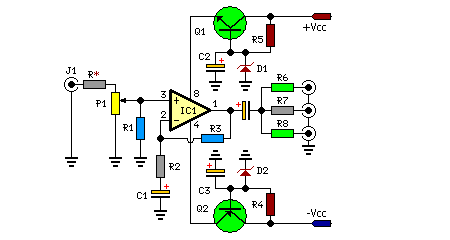# Learning Electronics

Learn to build electronic circuits

# Three Channel Audio Splitter

Simple circuitry, Passive high-quality amplification and distribution

This circuit is suitable to amplify and distribute the audio signals. The input audio signal is applied to the J1 and after passing through the P1, It is buffered and amplified by the IC1 prepared to redistribute. It has 3 outputs to drive 3 audio lines with 300 ohms impedance.

Circuit Diagram:Parts:

J1 = RCA Socket (See Notes)
P1 = 100K-Potentiometre
R* = 10K-100K
R1 = 560K
R2 = 1K
R3 = 2.2K
R4 = 2.7K
R5 = 2.7K
R6 = 330R
R7 = 330R
R8 = 330R
C1 = 100uF-25V
C2 = 100uF-25V
C3 = 100uF-25V
D1 = BZX79C18
D2 = BZX79C18
Q1 = BC337
Q2 = BC327
IC1 = NE5532-34

Notes:
• J1 will be RCA Audio input female socket.
• R* is on your choice it can be choose between 10K to 100K resistor.
• Output capacitor’s value is between 100uf to 470uf and power handling is 25V to 50V.
• You can power up this circuit via +12V/-12V regulated supply but you have to remove following parts Q1-Q2-C2-C3-D1-D2.
• Maximum power ratings +35V/-35V

Source : unknown